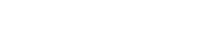# Certified Control Systems Technician (CCST) Question

• CCST

## CCST question

Each controller in the plant has instructions as part of the device that affects the controller output based on the error signal. These are preset based on the dynamic loop response and are referred to by which of the following terms:

A. algorithm
B. reverse band
D. error compensation

The answer is A, “algorithm.” An algorithm is a set of instructions or equations that are used to evaluate process inputs, such as sensor values, and determine the desired output. There are multiple algorithms that can be used. The familiar proportional, integral, derivative (PID) algorithm uses the difference between the sensor input and the set point (error) to calculate an output based on magnitude of the error, persistence of the error, and the rate of change of the error.

Reference: Goettsche, L. D. (Editor), Maintenance of Instruments and Systems, Second Edition, ISA, 2005.